# Tools for building flows

When designers reach for the 'flow' building app, many all too quickly reach for some kind of design tool. Illustrator, OmniGraffle, etc ... The fail here is that it is way to easy to slip into design mode when you are supposed to be thinking about UX, flow strategies and solving business problems. No one really cares that the flow chart is the most amazing thing they have ever seen.

## Graphviz - Graph Visualization Software

Graphviz is open source graph visualization software. Graph visualization is a way of representing structural information as diagrams of abstract graphs and networks. It has important applications in networking, bioinformatics, software engineering, database and web design, machine learning, and in visual interfaces for other technical domains.

Graphviz is pretty simple really. There is a downloadable app that you install to visualize your graph.

Building a graph using this tool has multiple benefits. One, when you are writing the code, you are logically thinking about the flow, not trying to design a good looking diagram. Second, this is a easy lesson in problem solving, pseudo code and algorithm building.

To create a graph, it uses the DOT language. You can use any text editor to create the file. The following are some examples of how you can simply build a graph with this tool.

## Syntax

### Graph types

#### Undirected graphs

At its simplest, DOT can be used to describe an undirected graph. An undirected graph shows simple relations between objects, such as friendship between people. The graph keyword is used to begin a new graph, and nodes are described within curly braces. A double-hyphen (--) is used to show relations between the nodes.

// The graph name and the semicolons are optional
graph graphname {
a -- b -- c;
b -- d;
}#### Directed graphs

Similar to undirected graphs, DOT can describe directed graphs, such as flowcharts and dependency trees. The syntax is the same as for undirected graphs, except the digraph keyword is used to begin the graph, and an arrow (->) is used to show relationships between nodes.

digraph graphname {
a -> b -> c;
b -> d;
}#### Attributes

Various attributes can be applied to graphs, nodes and edges in DOT files. These attributes can control aspects such as color, shape, and line styles. For nodes and edges, one or more attribute-value pairs are placed in square brackets ([]) after a statement and before the semicolon. Graph attribute are specified as direct attribute-value pairs under graph element. Multiple attributes are separated by a comma and a space. Node attributes are placed after a statement containing only the name of the node, and no relations.

graph graphname {
// This attribute applies to the graph itself
size="1,1";
// The label attribute can be used to change the label of a node
a [label="Foo"];
// Here, the node shape is changed.
b [shape=box];
// These edges both have different line properties
a -- b -- c [color=blue];
b -- d [style=dotted];
}## Illustrative examples

The following examples were copied from graphs.grevian.org

### Example 1: Simple Graph

graph {
a -- b;
b -- c;
a -- c;
d -- c;
e -- c;
e -- a;
}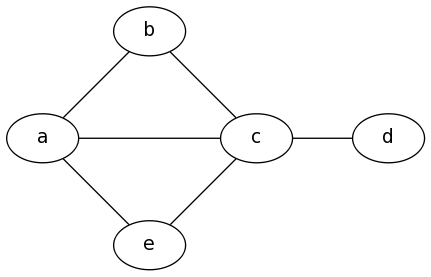### Example 2: K6

graph {
a -- b;
b -- c;
c -- d;
d -- e;
e -- f;
a -- f;
a -- c;
a -- d;
a -- e;
b -- d;
b -- e;
b -- f;
c -- e;
c -- f;
d -- f;
}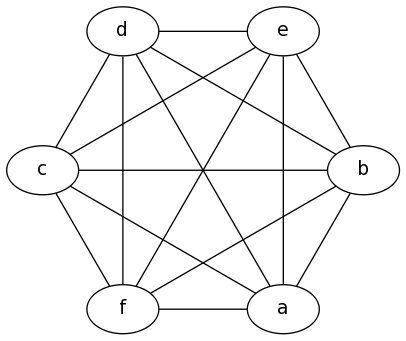### Example 3: Simple Digraph

digraph {
a -> b;
b -> c;
c -> d;
d -> a;
}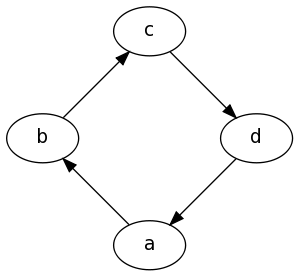### Example 4: Full Digraph

digraph {
a -> b[label="0.2",weight="0.2"];
a -> c[label="0.4",weight="0.4"];
c -> b[label="0.6",weight="0.6"];
c -> e[label="0.6",weight="0.6"];
e -> e[label="0.1",weight="0.1"];
e -> b[label="0.7",weight="0.7"];
}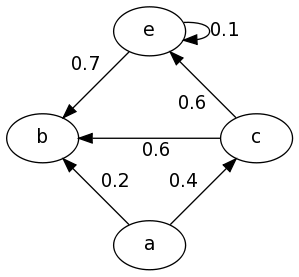### Example 5: Showing A Path

graph {
a -- b[color=red,penwidth=3.0];
b -- c;
c -- d[color=red,penwidth=3.0];
d -- e;
e -- f;
a -- d;
b -- d[color=red,penwidth=3.0];
c -- f[color=red,penwidth=3.0];
}


Note that there's also a shorthand method as follows:

graph {
a -- b -- d -- c -- f[color=red,penwidth=3.0];
b -- c;
d -- e;
e -- f;
a -- d;
}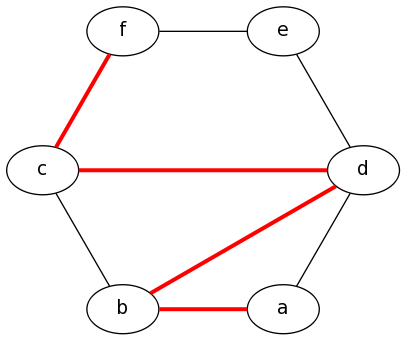### Example 6: Subgraphs

Please note there are some quirks here, First the name of the subgraphs are important, to be visually seperated they must be prefixed with cluster_ as shown below, and second only the DOT and FDP layout methods seem to support subgraphs (See the graph generation page for more information on the layout methods)

digraph {
subgraph cluster_0 {
label="Subgraph A";
a -> b;
b -> c;
c -> d;
}

subgraph cluster_1 {
label="Subgraph B";
a -> f;
f -> c;
}
}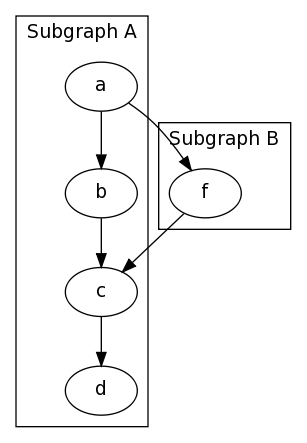Another Example of a Subgraph, In this example I group nodes together seperately from their edges, And also uses the graph attribute splines=line; to specify that edges should be drawn only as straight lines, no curves allowed.

graph {
splines=line;
subgraph cluster_0 {
label="Subgraph A";
a; b; c
}

subgraph cluster_1 {
label="Subgraph B";
d; e;
}

a -- e;
a -- d;
b -- d;
b -- e;
c -- d;
c -- e;
}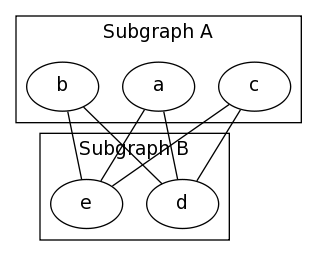### Example 7: Large Graphs

To make it easier to input large graph descriptions, One may group edges together with a set of braces, It may also help to lay the graph out left to right instead of top to bottom.

graph {
rankdir=LR; // Left to Right, instead of Top to Bottom
a -- { b c d };
b -- { c e };
c -- { e f };
d -- { f g };
e -- h;
f -- { h i j g };
g -- k;
h -- { o l };
i -- { l m j };
j -- { m n k };
k -- { n r };
l -- { o m };
m -- { o p n };
n -- { q r };
o -- { s p };
p -- { s t q };
q -- { t r };
r -- t;
s -- z;
t -- z;
}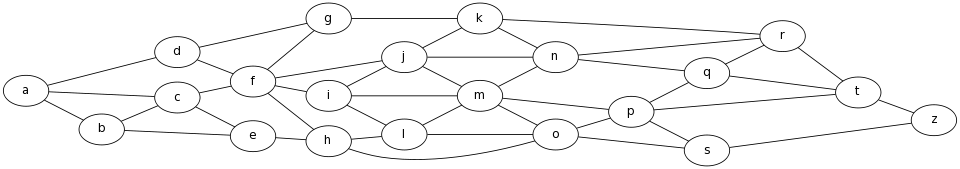Another feature that can make large graphs manageable is to group nodes together at the same rank, the graph above for example is copied from a specific assignment, but doesn't look the same because of how the nodes are shifted around to fit in a more space optimal, but less visually simple way. We can make it look much more similar by grouping the nodes together for display as is done in the assignment with rank, as follows

graph {
rankdir=LR;
a -- { b c d }; b -- { c e }; c -- { e f }; d -- { f g }; e -- h;
f -- { h i j g }; g -- k; h -- { o l }; i -- { l m j }; j -- { m n k };
k -- { n r }; l -- { o m }; m -- { o p n }; n -- { q r };
o -- { s p }; p -- { s t q }; q -- { t r }; r -- t; s -- z; t -- z;
{ rank=same, b, c, d }
{ rank=same, e, f, g }
{ rank=same, h, i, j, k }
{ rank=same, l, m, n }
{ rank=same, o, p, q, r }
{ rank=same, s, t }
}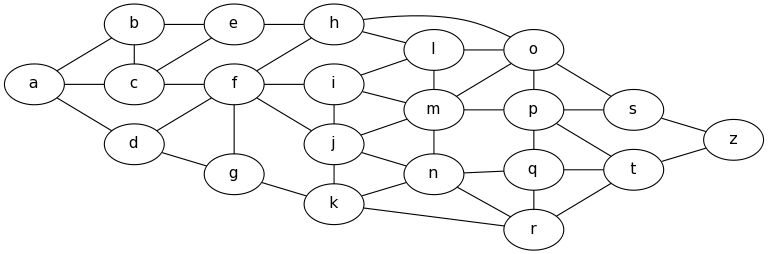## The whole API

Graphviz is an amazingly complex tool with a really rich API. Play with the options, view the gallery Hint: click on the full image to see the DOT code for that example.